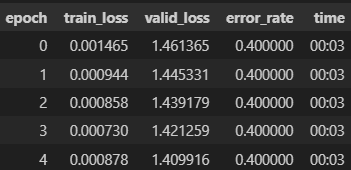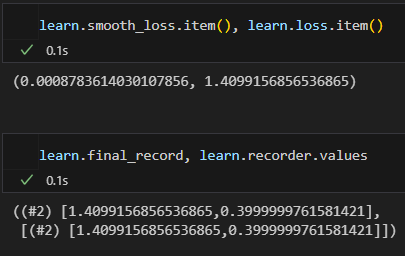# Learner. Validate method for train set

I try to use method validate of learner. And found a litle bit confusing thing.

There is a result of some training:When I use this code:

`learn.validate(0), learn.validate(1)`

I get this output

((#2) [0.00040706488653086126,0.0],
(#2) [1.4099156856536865,0.3999999761581421])

The second output is equal to the validate loss and error rate (for validate set I guess). But I don’t understand about the output of the `learn.validate(0)`. It is not equal to the `train_loss.`

.

.

Hi,

The method `validate` returns the average of the losses and the metric score for the inputed dataloader.
From the docs,

``````    def validate(self, ds_idx=1, dl=None, cbs=None):
if dl is None: dl = self.dls[ds_idx]
``````

[See https://github.com/fastai/fastai/blob/master/fastai/learner.py#L276C1-L277C45]
`learn.validate(0)` returns the average losses and the metric score for the train dataloader,
`learn.validate(1)` returns the average losses and the metric score for the test dataloader

The log however [i.e. the attached image above], prints out the smooth average of the losses as `train_loss`. [See the doc for the differences between the two averages: https://github.com/fastai/fastai/blob/master/fastai/learner.py#L490C1-L511C63 ].

It however prints the average of the losses as `valid_loss` which is expected.

One way to understand all of these is that while training a single epoch, the losses computed for each batch differs as the model updates its parameters thus, the representation used by fastai is the smooth average of these losses, but for validation there is no parameter update therefore the mean average of its losses is the better representation.

See the following commands;
`learn.smooth_loss.item()` → Returns the smooth average loss on the train dataloader
`learn.loss.item()` → Returns the average loss on the train dataloader

Try running these also;
`learn.final_record` → Should give the same output as `learn.validate(0)` without validating [I mean in O(1) and not O(n)].
`learn.recorder.values` → Same output

1 LikeIn my case `learn.loss.item()` return `valid_loss`.
As you may see it gives `valid_loss` in both cases.
Thank you for clarification! It really helped me. So, to get the true (not averaged on minibatches) `train_loss` I will use `learn.validate(0)`.